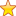# RunTime Polymorphism in C++

## C++ Runtime Polymorphism

As we all know, polymorphism is achieved by function overriding. The run time polymorphism in C++ is achieved greatly with the help of `virtual functions`, it happens largely due to multiple declaration of same functions in both base and derived classes.

It may be a little hard to understand this without any reference to any example. So let’s directly dig in into details.

### Definition issue with C++

Let us look at this example first to understand what is definition issue that is caused in C++ and later we will understand how `virtual functions` help us resolve this issue and create run time polymorphism.

```#include
using namespace std;

// This is Base class
class Base
{
public:
void show()
{
cout << "Showing Base Class" << endl;
}
};

// This is Derived class function
class Derived : public Base
{
public:

// This function is declared in the base class as well
void show()
{
cout << "Showing Derived Class" << endl;
}

};

int main()
{
// pointer reference to base class
Base *base_object;
// creating derived class object
Derived derived_object;
// mapping base object to address of derived class object
base_object = &derived_object;

// since we use pointers so we use -> rather than .
base_object->show();

return 0;
}```

Now, output is – Showing base class

• One would expect the derived class to be referred and print showing derived class
• It’s natural to think this way as the address referred finally is of the derived class right?
• But, this doesn’t happen, rather it prints showing base class

Now this happens because of how C++ is written and it causes something called as static call or static resolution which is a classic example of early binding.

The compiler sets the default function to be called at compile time itself and choses base class object and thus the derived class functions can never be called using pointed references between base and derived classes.

### How Run Time Polymorphism is achieved?

Polymorphism is achieved by function overriding, however, this problem of early binding at compile time is resolved by invoking run time polymorphism using virtual functions.

Let us understand this with an example on how this happens.

We add virtual keyword before the function in the base class.

```#include
using namespace std;
// This is Base class
class Base
{
public:
virtual void show()
{
cout << "Showing Base Class" << endl;
}
};
// This is Derived class function
class Derived : public Base
{
public:
// This function is declared in the base class as well
void show()
{
cout << "Showing Derived Class" << endl;
}
};

int main()
{
// pointer reference to base class
Base *base_object;
// creating derived class object
Derived derived_object;
// mapping base object to address of derived class object
base_object = &derived_object;
// since we use pointers so we use -> rather than .
base_object->show();
return 0;
}```

Output – Showing Derived Class

Now, using virtual keyword before the parent class declaration of the function we have asked compiler to not do early binding and only resolve this at run time. This is how, run time polymorphism is achieved in C++

Summary
Review Date
Reviewed Item
Runtime Polymorphism
Author Rating
5